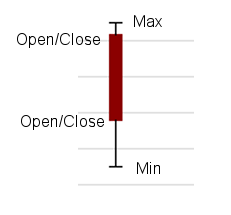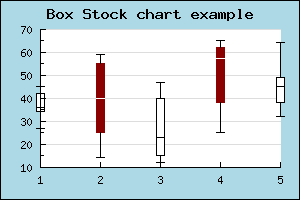## 15.4. Stock graphs

Stock charts are used to display data values where one is interested in 4 different values for each data point. This could for example be used to display a stocks open,close, min and max value during a specific day. Hence the name Stock chart (or Stock plot).

Stock plots are created as an instance of `class StockPlot` so the module "`jpgraph_stock.php`" must first be included in the script.

Figure 15.62illustrates a sample Stock chart plot

Figure 15.62. A stock graph `(stockex1.php)`For this type of plot the y-data array must be consist of a number of quadruples of data where each quadruple consists of (open,close,min,max). The open and close values determine the min max for the middle bar and the min,max determine the end points of the "error-lines" at the top and bottom of each bar as shown in ??.

 Figure 15.63. Explaining stock graphsFor this type of plot the y-data array must be consist of a number of quadruples of data where each quadruple consists of an even number of (open,close,min,max). The open and close values determine the min max for the middle bar and the min,max determine the end points of the "error-lines" at the top and bottom of each bar as shown in Figure 15.63. Note that the data follows the rules min < maxmin < min( open, close )max > max( open, close )

To separate the two cases where "open > close" or "open < close " two different colors are used.

These colors are specified with the method

• ```StockPlot::SetColor(\$aFrame, \$aFill='white', \$aFrameNeg='darkred', \$aFillNeg='darkred')```

`\$aFrame`, `\$aFill`, The frame and fill color when open <= close

`\$aFrameNeg`, `\$aFillNeg`, The frame and fill color when open > close

`StockPlot::SetColor()` method. By default a positive bar (close > open) have a fill color of white and for the negative case where (close < open) the bars have a red color.

The final possible variation of stock plots is the option to determine whether or not the end point for the min,max lines should have the horizontal line marking the end of the line or not. This can be adjusted with a call to method

• `StockPlot::HideEndLines(\$aHide=true)`

### 15.4.1. Stock plot variant: Boxplot

A minor variation of stock charts is the `BoxPlot` plot type. this is almost the same as `StockPlot` but with the very small difference that in addition to the open,close,min, max values it is also possible to specify a fifth, median value .

A box plot is created as in instance of `class BoxPlot` which is defined in "`jpgraph_stock.php`"

The median lies between the open and close value and is illustrated as a horizontal line within the bar. An example of this is shown in

Figure 15.64. A typical boxplot `(boxstockex1.php)`The color of the median line can be modified with a call to

• `BoxPlot::SetMedianColor(\$aColor)`

### 15.4.2.  Image maps for Box and Stock charts

In the same way as for other plots it is possible to associate an image map with these plots. The "hot" area for each plot is the mid "bar" section. In the same way as other plot types the method

• `BoxPlot::SetCSIMTargets(\$aTargets,\$aAlts='',\$aWinTargets='')`

is used to set the URL:s for the hot areas